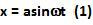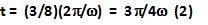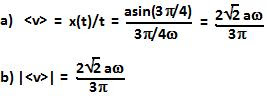## Saturday, May 29, 2010

### Irodov Problem 4.6

The equation of motion of the particle is given by,The time elapsed for 3/8 of the time period of oscillations is given by,As with problem 4.5, the mean velocity is the displacement per time and so we have,The mean of modulus of the velocity is essentially the mean speed of the particle and is given by the the total distance (not displacement) per time. As seen in the figure, in the first 2/8 (1/4) of the time period the particle travels a distance of a to reach the extreme position. After this point it reverses its journey and starts to move towards the center. Over the remaining 1/8 of the period, from (1) we can compute that the particle has reached a point that is distancefrom the center. This means that the particle has traveled a distanceduring the remaining 1/8 of the time period. The mean speed of the particle can thus be computed as,1.2.3.4.5.